### Example 8.3 Solving NLP Problems with Range Constraints

Some constraints have both lower and upper bounds (that is,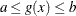). These constraints are called range constraints. The NLP solver can handle range constraints in an efficient way. Consider the following NLP problem, taken from Hock and Schittkowski (1981),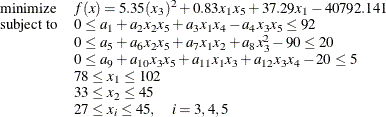where the values of the parameters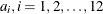, are shown in Table 8.4.

Table 8.4: Data for Example 3

 i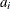ii1 85.334407 5 80.51249 9 9.300961 2 0.0056858 6 0.0071317 10 0.0047026 3 0.0006262 7 0.0029955 11 0.0012547 4 0.0022053 8 0.0021813 12 0.0019085

The initial point used is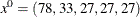. You can call the NLP solver within PROC OPTMODEL to solve this problem by writing the following statements:

proc optmodel;
number l {1..5} = [78 33 27 27 27];
number u {1..5} = [102 45 45 45 45];

number a {1..12} =
[85.334407 0.0056858 0.0006262 0.0022053
80.51249 0.0071317 0.0029955 0.0021813
9.300961 0.0047026 0.0012547 0.0019085];

var x {j in 1..5} >= l[j] <= u[j];

minimize f = 5.35*x^2 + 0.83*x*x + 37.29*x
- 40792.141;

con constr1:
0 <= a + a*x*x + a*x*x -
a*x*x <= 92;
con constr2:
0 <= a + a*x*x + a*x*x +
a*x^2 - 90 <= 20;
con constr3:
0 <= a + a*x*x + a*x*x +
a*x*x - 20 <= 5;

x = 78;
x = 33;
x = 27;
x = 27;
x = 27;

solve with nlp / algorithm=activeset;
print x;
quit;


The summaries and solution are shown in Output 8.3.1.

Output 8.3.1: Summaries and the Optimal Solution

The OPTMODEL Procedure

Problem Summary
Objective Sense Minimization
Objective Function f

Number of Variables 5
Bounded Above 0
Bounded Below 0
Bounded Below and Above 5
Free 0
Fixed 0

Number of Constraints 3
Linear LE (<=) 0
Linear EQ (=) 0
Linear GE (>=) 0
Linear Range 0
Nonlinear LE (<=) 0
Nonlinear EQ (=) 0
Nonlinear GE (>=) 0
Nonlinear Range 3

Performance Information
Execution Mode Single-Machine
Number of Threads 4

Solution Summary
Solver NLP
Algorithm Active Set
Objective Function f
Solution Status Optimal
Objective Value -30689.16932

Optimality Error 2.2010098E-8
Infeasibility 0

Iterations 20
Presolve Time 0.00
Solution Time 0.04

 x
1 78.000
2 33.000
3 29.995
4 45.000
5 36.776